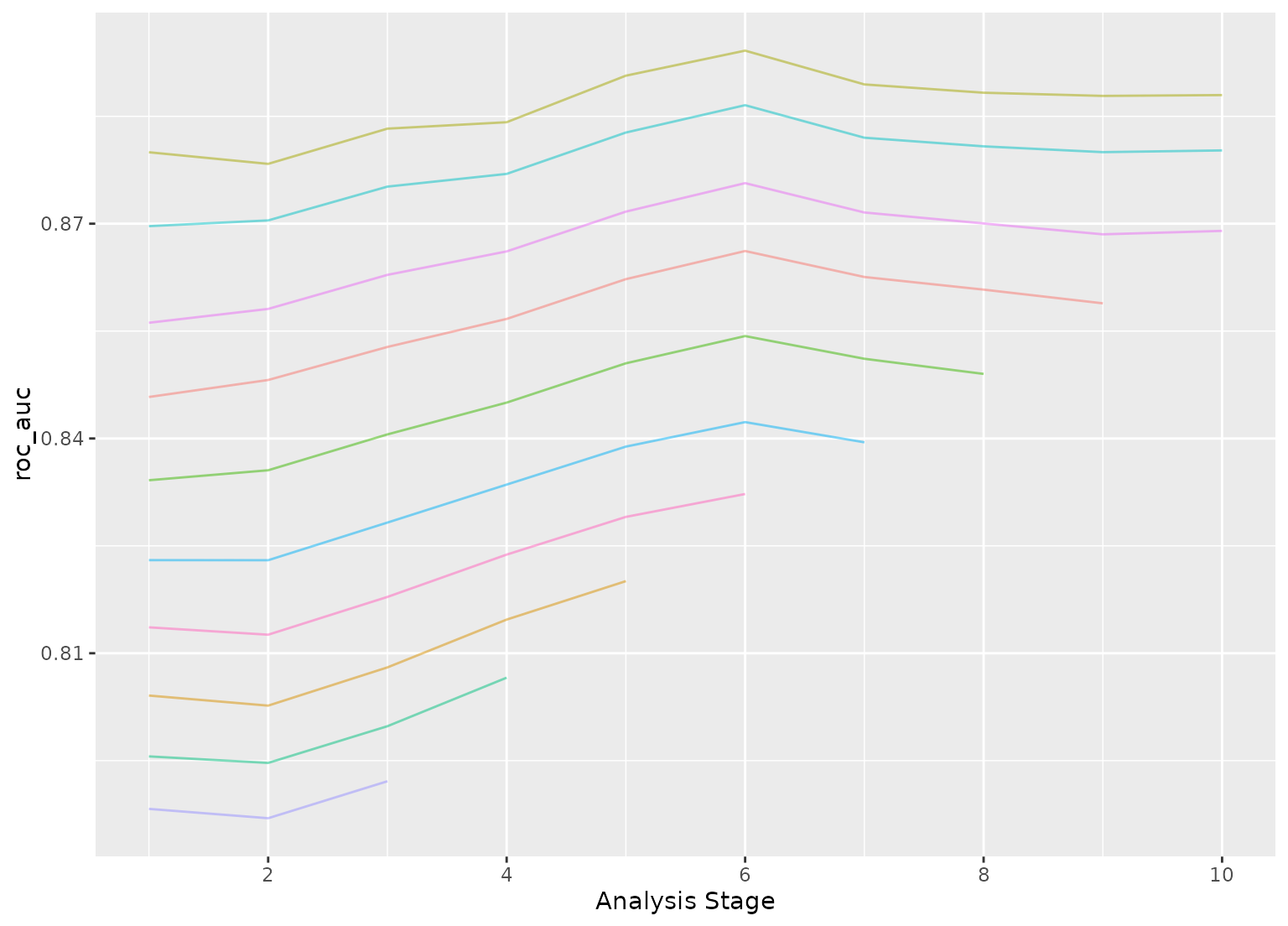tune_race_win_loss() computes a set of performance metrics (e.g. accuracy or RMSE) for a pre-defined set of tuning parameters that correspond to a model or recipe across one or more resamples of the data. After an initial number of resamples have been evaluated, the process eliminates tuning parameter combinations that are unlikely to be the best results using a statistical model. For each pairwise combinations of tuning parameters, win/loss statistics are calculated and a logistic regression model is used to measure how likely each combination is to win overall.

tune_race_win_loss(object, ...)

# S3 method for model_spec
tune_race_win_loss(
object,
preprocessor,
resamples,
...,
param_info = NULL,
grid = 10,
metrics = NULL,
control = control_race()
)

# S3 method for workflow
tune_race_win_loss(
object,
resamples,
...,
param_info = NULL,
grid = 10,
metrics = NULL,
control = control_race()
)

## Arguments

object A parsnip model specification or a workflows::workflow(). Not currently used. The technical details of this method are described in Kuhn (2014). Racing methods are efficient approaches to grid search. Initially, the function evaluates all tuning parameters on a small initial set of resamples. The burn_in argument of control_race() sets the number of initial resamples. The performance statistics from the current set of resamples are converted to win/loss/tie results. For example, for two parameters (j and k) in a classification model that have each been resampled three times:  | area under the ROC curve | ----------------------------- resample | parameter j | parameter k | winner --------------------------------------------- 1 | 0.81 | 0.92 | k 2 | 0.95 | 0.94 | j 3 | 0.79 | 0.81 | k ---------------------------------------------  After the third resample, parameter k has a 2:1 win/loss ratio versus j. Parameters with equal results are treated as a half-win for each setting. These statistics are determined for all pairwise combinations of the parameters and a Bradley-Terry model is used to model these win/loss/tie statistics. This model can compute the ability of a parameter combination to win overall. A confidence interval for the winning ability is computed and any settings whose interval includes zero are retained for future resamples (since it is not statistically different form the best results). The next resample is used with the remaining parameter combinations and the statistical analysis is updated. More candidate parameters may be excluded with each new resample that is processed. The control_race() function contains are parameter for the significance cutoff applied to the Bradley-Terry model results as well as other relevant arguments. A traditional model formula or a recipe created using recipes::recipe(). An rset() object that has multiple resamples (i.e., is not a validation set). A dials::parameters() object or NULL. If none is given, a parameters set is derived from other arguments. Passing this argument can be useful when parameter ranges need to be customized. A data frame of tuning combinations or a positive integer. The data frame should have columns for each parameter being tuned and rows for tuning parameter candidates. An integer denotes the number of candidate parameter sets to be created automatically. A yardstick::metric_set() or NULL. An object used to modify the tuning process.

Kuhn, M 2014. "Futility Analysis in the Cross-Validation of Machine Learning Models." https://arxiv.org/abs/1405.6974.

## See also

tune::tune_grid(), control_race(), tune_race_anova()

## Examples

# \donttest{
library(parsnip)
library(rsample)
library(discrim)
library(dials)

## -----------------------------------------------------------------------------

data(two_class_dat, package = "modeldata")

set.seed(6376)
rs <- bootstraps(two_class_dat, times = 10)

## -----------------------------------------------------------------------------

# optimize an regularized discriminant analysis model
rda_spec <-
discrim_regularized(frac_common_cov = tune(), frac_identity = tune()) %>%
set_engine("klaR")

## -----------------------------------------------------------------------------

ctrl <- control_race(verbose_elim = TRUE)

set.seed(11)
grid_wl <-
rda_spec %>%
tune_race_win_loss(Class ~ ., resamples = rs, grid = 10, control = ctrl)
#> ℹ Racing will maximize the roc_auc metric.#> ℹ Resamples are analyzed in a random order.#> ℹ Bootstrap05:  1 eliminated;  9 candidates remain.#> ℹ Bootstrap07:  1 eliminated;  8 candidates remain.#> ℹ Bootstrap10:  1 eliminated;  7 candidates remain.#> ℹ Bootstrap01:  1 eliminated;  6 candidates remain.#> ℹ Bootstrap08:  1 eliminated;  5 candidates remain.#> ℹ Bootstrap03:  1 eliminated;  4 candidates remain.#> ℹ Bootstrap09:  1 eliminated;  3 candidates remain.
# Shows only the fully resampled parameters
show_best(grid_wl, metric = "roc_auc")
#> # A tibble: 3 x 8
#>   frac_common_cov frac_identity .metric .estimator  mean     n std_err .config
#>             <dbl>         <dbl> <chr>   <chr>      <dbl> <int>   <dbl> <chr>
#> 1          0.0691        0.0437 roc_auc binary     0.886    10 0.00513 Preproce…
#> 2          0.392         0.154  roc_auc binary     0.877    10 0.00496 Preproce…
#> 3          0.555         0.293  roc_auc binary     0.863    10 0.00524 Preproce…
plot_race(grid_wl)# }